# Thread: Integrals help!

1. ## Integrals help!

Hi,

Q1. Evaluate the following integrals by using the given substitution.

f [{e^squareroot(x)}/{2*squareroot(x)}], substitution u = squareroot(x)

Could someone please direct the topic about the question?

thanks

2.Originally Posted by Moon HopliteHi,

Q1. Evaluate the following integrals by using the given substitution.

f [{e^squareroot(x)}/{2*squareroot(x)}], substitution u = squareroot(x)

Could someone please direct the topic about the question?

thanks
$\displaystyle \int \frac{e^{\sqrt{x}}}{2\sqrt{x}}~dx$

Subbing $\displaystyle u = \sqrt{x} \implies du = \frac{1}{2\sqrt{x}}~dx$ switches the integral to:
$\displaystyle \int e^u~du$

And I'm sure you know how to find it now.

3. sorry but i dont understand anything from the question... could u please direct a topic for me to research and learn?

thanks

4. Are you studying this at the moment, I would suggest asking you tutor/teacher to go over it with you.

Searching google and youtube can also be helpful:

YouTube - integration by substitution

integration by substitution - Google Search

5. And, if a picture helps...

If a substitution is suggested you're probably working backwards through the chain rule for differentiation, which we can draw like this...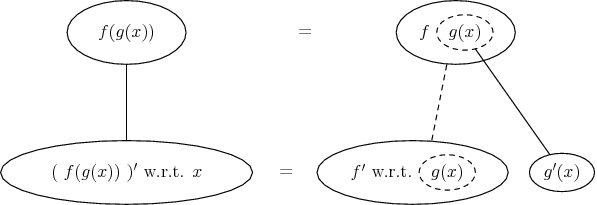...where straight continuous lines differentiates downwards with respect to x, and the dashed straight line with respect to the dashed balloon expression. So you can see your solution here...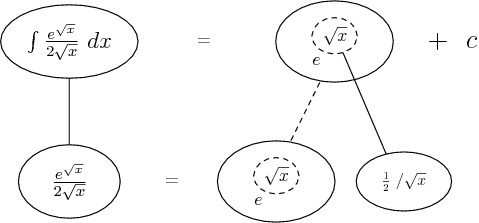Start by identifying the inner function...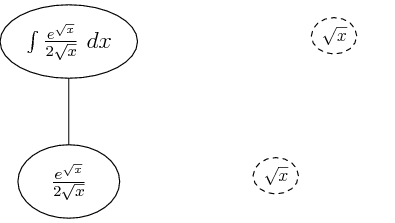Differentiate it for the sake of the chain rule...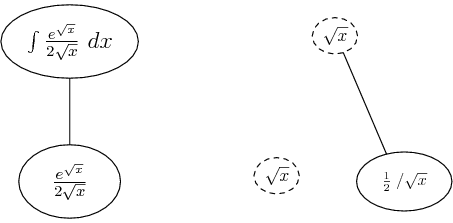Make sure the lower level is equal to what you want to integrate...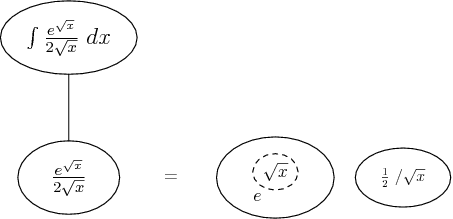Then you can integrate as though the dashed balloon were a variable...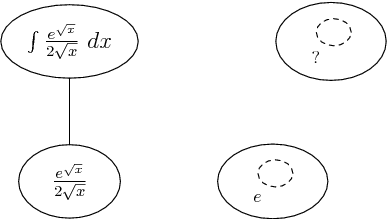Don't integrate - balloontegrate! Balloon Calculus: worked examples from past papers

#### Search Tags

integrals